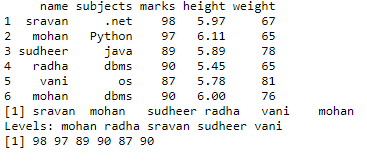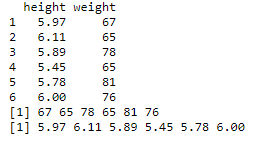Open In App

# Extract vector from dataframe in R

In this article, we will see how to extract vectors from DataFrame in R Programming Language.

The approach is simple, by using \$ operator, we can convert dataframe column to a vector.

Syntax:

dataframe_name\$columnname

Given below are various examples to implement the same

Example 1:

## R

 `# create vector with names``name=``c``(``"sravan"``,``"mohan"``,``"sudheer"``,``"radha"``,``"vani"``,``"mohan"``)`` ` `# create vector with subjects``subjects=``c``(``".net"``,``"Python"``,``"java"``,``"dbms"``,``"os"``,``"dbms"``)`` ` `# create a vector with marks``marks=``c``(98,97,89,90,87,90)`` ` `# create vector with height``height=``c``(5.97,6.11,5.89,5.45,5.78,6.0)`` ` `# create vector with weight``weight=``c``(67,65,78,65,81,76)`` ` `# pass these vectors to the data frame``data=``data.frame``(name,subjects,marks,height,weight)`` ` `# display dataframe``print``(data)`` ` `# access vector from dataframe column name``a=(data\$name)``print``(a)`` ` `# access vector from dataframe column marks``b=(data\$marks)``print``(b)`

Output:Example 2:

## R

 `# create vector with height``height=``c``(5.97,6.11,5.89,5.45,5.78,6.0)`` ` `# create vector with weight``weight=``c``(67,65,78,65,81,76)`` ` `# pass these vectors to the data frame``data=``data.frame``(height,weight)`` ` `# display dataframe``print``(data)`` ` `# access vector from dataframe column``# weight``a=(data\$weight)``print``(a)`` ` `# access vector from dataframe column ``# height``b=(data\$height)``print``(b)`

Output: